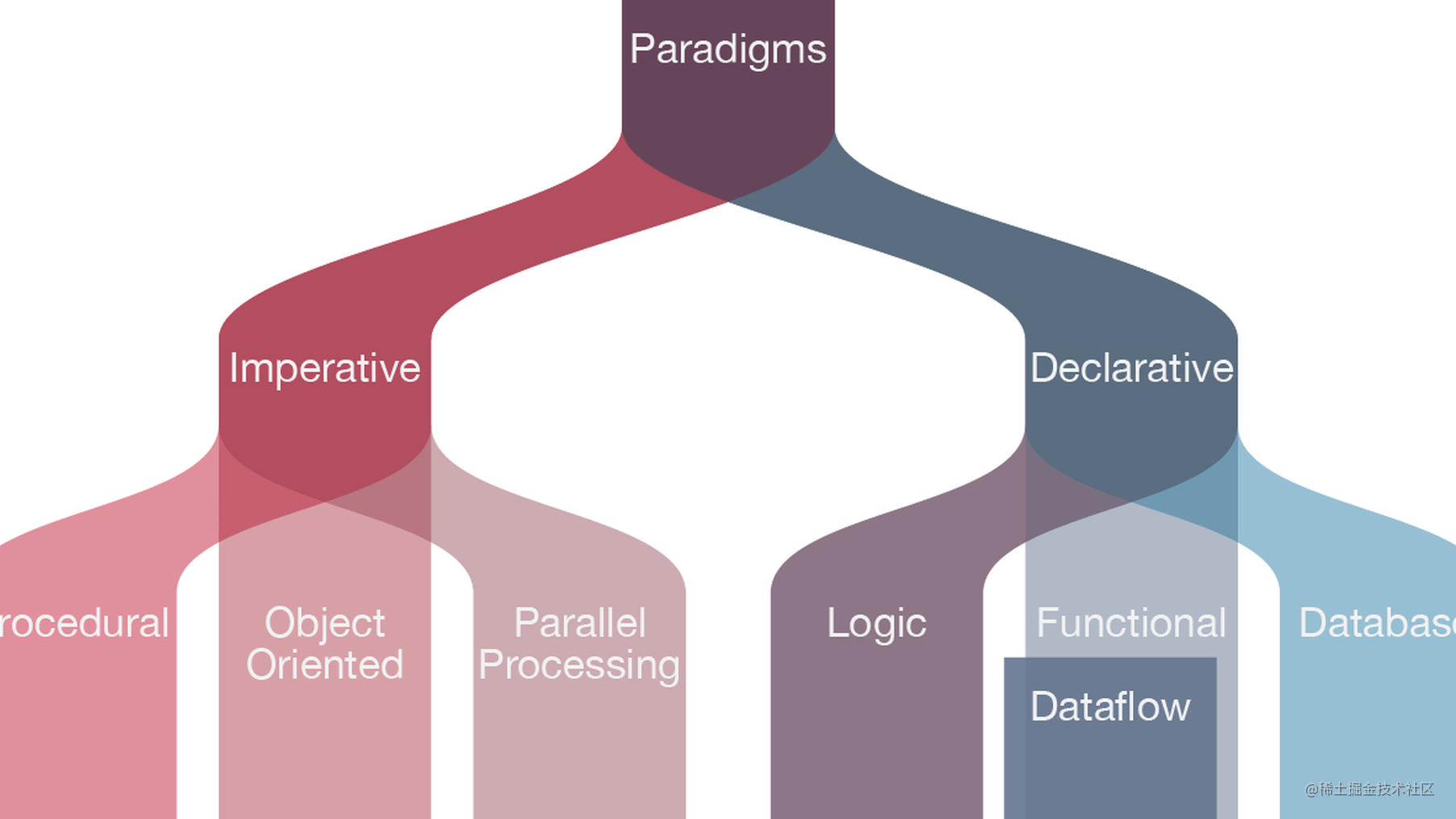编写扁平化的代码-- 给你的代码增加一点点函数式编程的特性

循环

...一个循环就是一个命令式控制结构，难以重用，并且难以插入到其他操作中。此外，它还得不断变化代码来响应新的迭代需求。
-- Luis Atencio

function helloworld(arr) {
for (let i = 1; i < arr.length; i++) {
arr[i] *= 2
if (arr[i] % 2 === 0) {
doSomething(arr[i])
}
}
}

function helloworld(arr) {
const evenNumbers = n => n % 2 === 0

arr
.slice(1)
.map(v => v * 2)
.filter(evenNumbers)
.forEach(v => doSomething(v))
}

[
{
"country": "NL",
"points": 12
},
{
"country": "BE",
"points": 3
},
{
"country": "NL",
"points": 0
},
...
]

let score = 0;

for (let i = 0; i < votes.length; i++) {
}
}

return score;
}

const sum = (a, b) => a + b;

.filter(vote => vote.country === 'NL')
.map(vote => vote.points)
.reduce(sum);
}

if else 语句

// Block 1
if (condition) {
doThis();
} else {
doThat();
}

// Block 2
const value = condition ? doThis() : doThat();

if (condition) {
const a = 'foo';
} else {
const a = 'bar';
}

const b = condition ? 'foo' : 'bar';

console.log(a); // Uncaught ReferenceError: a is not defined
console.log(b); // 'bar'

const box = element.getBoundingClientRect();

if (box.top - document.body.scrollTop > 0 && box.bottom - document.body.scrollTop < window.innerHeight) {
reveal();
} else {
hide();
}

const box = element.getBoundingClientRect();
const isInViewport =
box.top - document.body.scrollTop > 0 &&
box.bottom - document.body.scrollTop < window.innerHeight;

isInViewport ? reveal() : hide();

elements
.forEach(element => {
const box = element.getBoundingClientRect();

if (box.top - document.body.scrollTop > 0 && box.bottom - document.body.scrollTop < window.innerHeight) {
reveal();
} else {
hide();
}

});

const isInViewport = element => {
const box = element.getBoundingClientRect();
const topInViewport = box.top - document.body.scrollTop > 0;
const bottomInViewport = box.bottom - document.body.scrollTop < window.innerHeight;
};

elements
.forEach(elem => isInViewport(elem) ? reveal() : hide());

import { isInViewport } from 'helpers';

elements
.forEach(elem => isInViewport(elem) ? reveal() : hide());

import { passwordRegex as requiredChars } from 'regexes'
import { getJson } from 'helpers'

const validatePassword = async value => {
if (value.length < 6) return false
if (!requiredChars.test(value)) return false

if (forbidden.includes(value)) return false

return value
}

import { minLength, matchesRegex, notBlacklisted } from 'validationRules'
import { passwordRegex as requiredChars } from 'regexes'
import { getJson } from 'helpers'

const validatePassword = async value => {
const result = Array.from(value)
.filter(minLength(6))
.filter(matchesRegex(requiredChars))
.shift()

if (result) return result
throw new Error('something went wrong...')
}

import { minLength, matchesRegex, notBlacklisted } from 'validationRules'
import { passwordRegex as requiredChars } from 'regexes'
import { getJson } from 'helpers'

const validatePassword = async value =>
value
|> minLength(6)
|> matchesRegex(requiredChars)

try { someValue |> await validatePassword |> persist }
catch(e) {
// handle specific error, thrown in validation rule
}

事件

import { apiCall } from 'helpers'

class AutoComplete {

constructor (options) {

this._endpoint = options.endpoint
this._threshold = options.threshold
this._inputElement = options.inputElement
this._containerElement = options.list

this._onInput())

}

_onInput () {

const value = this._inputElement.value

if (value > this._options.threshold) {
this._updateList(value)
}

}

_updateList (value) {

apiCall(this._endpoint, { value })
.then(items => this._render(items))
.then(html => this._containerElement = html)

}

_render (items) {

let html = ''

items.forEach(item => {
html += `<a href="\${ item.href }">\${ item.label }</a>`
})

return html

}

}

Observable 类型可用于基于推送模型的数据源，如 DOM 事件，定时器和套接字

Observable 提案目前处于 Stage-1。在下面 listen 函数的实现是从 GitHub 上的提案中直接复制的，主要是将事件监听器转换成 Observable。可以看到，我们可以将整个 AutoComplete 类重写为单个方法的函数链。

import { apiCall, listen } from 'helpers';
import { renderItems } from 'templates';

function AutoComplete ({ endpoint, threshold, input, container }) {

listen(input, 'input')
.map(e => e.target.value)
.filter(value => value.length >= threshold)
.forEach(value => apiCall(endpoint, { value }))
.then(items => renderItems(items))
.then(html => container.innerHTML = html)

}

--

The flatter the better!